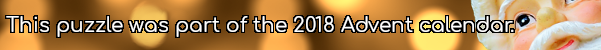mscroggs.co.uk
mscroggs.co.uksubscribe

# Puzzles

## 2 DecemberToday's number is the area of the largest dodecagon that it's possible to fit inside a circle with area $$\displaystyle\frac{172\pi}3$$.

## Cube multiples

Six different (strictly) positive integers are written on the faces of a cube. The sum of the numbers on any two adjacent faces is a multiple of 6.
What is the smallest possible sum of the six numbers?

## Polygraph

Draw a regular polygon. Connect all its vertices to every other vertex. For example, if you picked a pentagon or a hexagon, the result would look as follows:
Colour the regions of your shape so that no two regions which share an edge are the same colour. (Regions which only meet at one point can be the same colour.)
What is the least number of colours which this can be done with?

## Archive

Show me a random puzzle
▼ show ▼